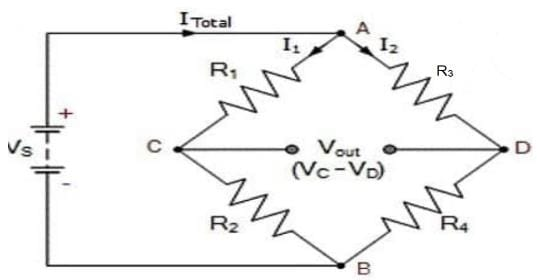JEE  >  Test: Kirchoff's Laws

# Test: Kirchoff's Laws

Test Description

## 5 Questions MCQ Test Physics Class 12 | Test: Kirchoff's Laws

Test: Kirchoff's Laws for JEE 2023 is part of Physics Class 12 preparation. The Test: Kirchoff's Laws questions and answers have been prepared according to the JEE exam syllabus.The Test: Kirchoff's Laws MCQs are made for JEE 2023 Exam. Find important definitions, questions, notes, meanings, examples, exercises, MCQs and online tests for Test: Kirchoff's Laws below.
Solutions of Test: Kirchoff's Laws questions in English are available as part of our Physics Class 12 for JEE & Test: Kirchoff's Laws solutions in Hindi for Physics Class 12 course. Download more important topics, notes, lectures and mock test series for JEE Exam by signing up for free. Attempt Test: Kirchoff's Laws | 5 questions in 10 minutes | Mock test for JEE preparation | Free important questions MCQ to study Physics Class 12 for JEE Exam | Download free PDF with solutions
 1 Crore+ students have signed up on EduRev. Have you?
Test: Kirchoff's Laws - Question 1

### At any junction, the sum of the currents entering the junction is equal to the sum of _______

Detailed Solution for Test: Kirchoff's Laws - Question 1

Kirchhoff's Current Law or KCL, states that the “total current or charge entering a junction or node is exactly equal to the charge leaving the node as it has no other place to go except to leave, as no charge is lost within the node“. In other words the algebraic sum of ALL the currents entering and leaving a node must be equal to zero, I(exiting) + I(entering) = 0.

Test: Kirchoff's Laws - Question 2

### The ______ of changes in potential around any closed loop involving resistors and cells in a loop is zero.

Detailed Solution for Test: Kirchoff's Laws - Question 2

In accordance with Kirchhoff’s second law i.e. Kirchhoff’s voltage law (KVL), the algebraic sum of all the potential differences in a closed electric circuit or closed loop that contains one or more cells and resistors is always equal to zero.
This law is popularly called the law of conservation of voltage.

Test: Kirchoff's Laws - Question 3

### The Wheatstone bridge Principle is deduced using

Detailed Solution for Test: Kirchoff's Laws - Question 3

Kirchoff's Law states that the algebraic sum of currents at a junction of an electric circuit is zero. ... Wheatstone bridge is formed by connecting a battery B or an electric source, a plug key K and a variable resistor X between the junctions A and D and a galvanometer G between the junctions C and D.Test: Kirchoff's Laws - Question 4

Given N resistors each of resistance R are first combined to get minimum possible resistance and then combined to get maximum possible resistance. The ratio of the minimum to maximum resistance is​

Detailed Solution for Test: Kirchoff's Laws - Question 4

Minimum possible resistance can be found in a parallel arrangement and maximum possible resistance can be found in a series arrangement.
Thus, 1/Rmin=1/R+1/R+....+ n times
=n/R
⇒Rmin=R/n
and Rmax=R+R+.......+ n times
⇒Rmax=nR
∴Rmin/Rmax=(R/n)/nR=1/n2

Test: Kirchoff's Laws - Question 5

Two cells of 1.25 V and 0.75V are connected in parallel. The effective voltage will be

Detailed Solution for Test: Kirchoff's Laws - Question 5

When two cells are conneted in parallel , then

Effective Voltage,V = V1 − V2 = 1.25 − 0.75 = 0.5 V

## Physics Class 12

157 videos|452 docs|213 tests
 Use Code STAYHOME200 and get INR 200 additional OFF Use Coupon Code
Information about Test: Kirchoff's Laws Page
In this test you can find the Exam questions for Test: Kirchoff's Laws solved & explained in the simplest way possible. Besides giving Questions and answers for Test: Kirchoff's Laws, EduRev gives you an ample number of Online tests for practice

## Physics Class 12

157 videos|452 docs|213 tests